# An Adaptive Gradient Boosting Model for the Prediction of Rainfall Using ID3 as a Base Estimator

An Adaptive Gradient Boosting Model for the Prediction of Rainfall Using ID3 as a Base Estimator
Department of Computer Sciences, University of Kashmir, J&K 190006, India

Directorate of IT&SS, University of Kashmir, J&K 190006, India

Corresponding Author Email:
zamanmajid@gmail.com
Page:
241-250
|
DOI:
https://doi.org/10.18280/ria.360208
16 December 2021
|
Accepted:
4 April 2022
|
Published:
30 April 2022
| Citation

OPEN ACCESS

Abstract:

While analyzing the data, it is crucial to choose the model that best matches the circumstance. Many experts in the field of classification and regression have proposed ensemble strategies for tabular data, as well as various approaches to classification and regression problems. In this paper, Gini Index is applied on raw geographical dataset to convert continuous data into discrete dataset. Decision tree algorithm is implemented on resultant discrete dataset, Information Gain is calculated for every attribute and the attribute with highest information gain is the splitting node, applied recursively. Decision tree algorithm implemented predicts the rainfall in Kashmir province with the accuracy of 81.5%. MDL pruning is applied on the resultant decision tree in order to reduce the size & complexity of the Decision tree. Pruning removes segments of the tree that contribute little towards classification; the accuracy is marginally reduced to 81.1%. Furthermore, after the implementation of Decision tree a boosting algorithm: gradient boosting has been implemented on the same set of data using decision tree as a base estimator. It was observed that the overall accuracy of the decision tree got increased to 87.5% after the implementation of gradient boosting model. Thus, the obtained results predict that gradient boosted-DT outperforms all other approaches with the highest accuracy measure and high susceptibility rate in rainfall prediction.

Keywords:

decision tree, Gini index, geographical data mining, information gain, gradient boosting

1. Introduction

Machine learning is learning from data, accordingly machine learning is design and implementation of models of data, mostly mathematical. Once the models have been trained and tested on existing datasets, these models are used to make predictions on new datasets. Machine learning is broadly classified into supervised and unsupervised learning.

Supervised learning is determining relationship between features of data with its associated label, once this relationship is established, we have model in place, and the resultant model is applied to predict label/s of new datasets. Supervised learning is of two types, classification and regression [1, 2]. Classification is two-step mechanism, learning phase-which results in classification model and classification phase-where model is applied to make prediction. Classification has numerous applications in our day-to-day life; this includes medical science, academics, automobile industry, geography etc. Decision tree classifier is one of the most popular classifiers [3, 4].

1.1 Decision tree

ID3 known as C4.5, and this continues to be benchmark algorithm till date. In mid-1980’s Classification and Regression Tree (CART) algorithm was proposed by L. Breiman and other, CART describes generation of binary tree. ID3, C4.5 and CART construct decision tree using recursive divide and conquer method. The implementation of decision tree requires training dataset (tuples with labels), which is recursively partitioned into smaller subsets till no further partitions are required or are possible.

A decision tree is a tree like structure, which has the root node, internal node(s), branch(s) and leaf node(s). The top most node is the root node and it is also the starting point of the decision tree, while as internal node represents a test/condition on the given attribute; each branch coming out of node represents a possible outcome of the test/condition while as leaf node is the final outcome and holds the class label .

The creation of decision tree classifier is void of any dominion knowledge understanding, thus are suitable for empirical knowledge discovery. Decision tree can be implemented on multidimensional data. Decision tree are easy to understand and implement besides their representation is natural to human understanding. The decision tree is supervised learning and has learning and classification steps which are simple to implement and exhibits fast performance. The accuracy of classifier is data specific and thus varies from model to model however in general decision tree classifiers have shown good accuracy. Attribute selection measures are central to the design and implementation of decision tree; attribute selection is the process of selecting attributes that best partitions the data into distinct classes . The resultant decision tree may have branches that reflect noise or outliers in the training data set. The methodology of identifying and removing such branches is known as pruning, the purpose of such removal/s is improvisation of accuracy. Since, we are generating an immeasurable amount of data. It has become a need to develop more advanced and complex machine learning techniques. Boosting machine learning is one such technique that can be used to solve complex data driven real-world problems .

In order to solve complex and convoluted problems we require more advanced techniques. Boosting is a type of ensemble learning technique that uses a set of machine learning algorithms in order to convert or combine weak learners to strong learners so that the accuracy of one model gets increased. Boosting is actually a very effective method in order to increase the efficiency of the model.

## 1.jpg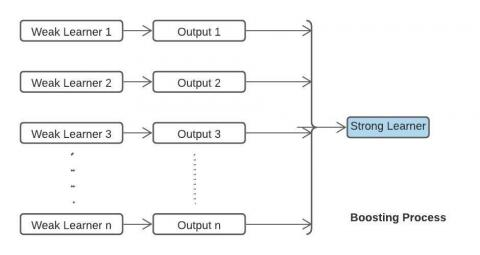Figure 1. Boosting process

Above figure (Figure 1) shows the basic principle of boosting process by combining the outputs that we get from all the weak learners in order to get a strong learner.

Gradient Boosting is based on a sequential ensemble learning model for both classification and regression problems in which the base learners are generated sequentially in such a way that the present base learner is always more effective than previous one. Basically, the overall model improves sequentially with each iteration. In this type of boosting the weights for misclassified outcomes are not incremented i.e. we are not going to add weights to misclassified outcomes instead in gradient boosting we try to optimize the loss function (residual) of the previous learner by adding a new adaptive model that adds weak learners in order to reduce the loss function. The main idea of gradient boosting is to overcome the errors in the previous learner’s prediction.

Since then, a great deal of research has been done using traditional and ensemble algorithms to predict rainfall, but no single algorithm can predict accurately regardless of the dataset. As a result, the primary goal of this paper is to propose a benchmark algorithm that will perform well across a wide range of datasets.

2. Literature Review

In this paper, a brief review is provided to summarize the recent studies on the weather forecasting mostly - Rainfall prediction [8-12] using various data mining classification techniques.

In  the study , a comparison has been made on the research done by different authors on weather prediction in which Hemalatha  implements decision tree technique using C4.5 and Iterative Dicotomizer 3 (ID3) algorithm on 20 – 30 instances of previous data and Petre  implements Decision tree technique using CART algorithm on 48 instances of data available from 2002 - 2005 and Ji et al. , Dept. of Computer Science, Bowie State University, implements Decision tree technique using C4.5, CART algorithms on previous data available consisting of 26280 instances. It was observed that the Decision tree in individual preforms better accuracy of 93 – 99% when compared with other algorithms.

Fayaz et al.  have proposed an experimental evaluation of Information gain and Gini Index for the construction of Decision trees. The dataset used in this study was collected from Indian Metrological department Pune, India. The parameters include temperature (minimum, maximum), Humidity (3 P.M and 12 A.M), season (summer, winter, Autumn & Spring) and the binary target variable with Yes or No values. The study constructs the decision tree based on information gain and GINI index and based on the rules of each decision tree the accuracy measure was calculated. It was observed that the information gain based on the dataset used performs well as compared to Gini index.

In Ref. , an analytical comparison has been made between information gain and Gini Index for the construction of decision tree. The authors worked on two splitting criteria’s where the same dataset which was used as in the study . It was observed that the information gain and Gini index show head to head same values at many attributes but there are some variable present which shows different values. This comparison study describes the efficiency of both splitting criteria’s. Latter on the continuous dataset was then labeled using GINI index where the attributes were divided into binary values based on the values obtained from GINI index.

Fayaz et al.  worked on the same set of labeled data which was used in . The study defines the application of ensemble distributed decision tree for the prediction of rainfall. In this study, the dataset was divided into 3 parts based on the station id. The individual decision trees were constructed and the performance of each decision tree was calculated. Based on the voting approach of the three smaller decision trees a final accuracy was calculated. Furthermore, the final accuracy was then compared with the original decision tree accuracy. It was observed that the distributed decision tree accuracy is reduced considerably then the accuracy measure of original decision tree.

Moreover, in Refs. [20-23] many network models were proposed for monthly rainfall rate forecasting and climate changes. The performance of these proposed models was turned quite effective. The experiment results predict the better accuracy rates.

Since then, many authors have applied a variety of traditional and ensembled techniques to the tabular dataset to predict rainfall, and some of them are mentioned in this study. We can conclude from the literature that there is no single algorithm that performs better on different types of datasets. On one dataset that they used in their paper, many researchers predicted the accuracy of the proposed algorithms. As a result, the goal of this paper is to propose a benchmark algorithm that will perform well on a variety of datasets.

3. Dataset

For the implementation process in this paper, we used the discretized dataset obtained from , where the weather parameters in this dataset are taken from three different regions of Kashmir division, including the Northern part—Gulmarg area with station ID of 42026, the Southern part – Qazigund area with station ID of 42027, and the Central part – Srinagar area with station ID of 42044, and this data was collected from the Indian Metrological Department (IMD) Pune, India. The dataset used by Kaul et al.  is from the Kashmir region of India, and it contains around 7000 records in two CSV files from the years 2012 to 2017.

The snapshots of the dataset shown below, consists all the attributes which are used in the implementation of the decision tree. These datasets include relative humidity which has been measured in % at 12 AM and 3PM for all three regions of Kashmir division (Figure 2). There are around 12190 instances of relative humidity and 6117 instances of other attributes including Maximum temperature (°C), Rainfall (in mm) and Minimum temperature (°C) for all the three stations, (Figure 3).

## 2.png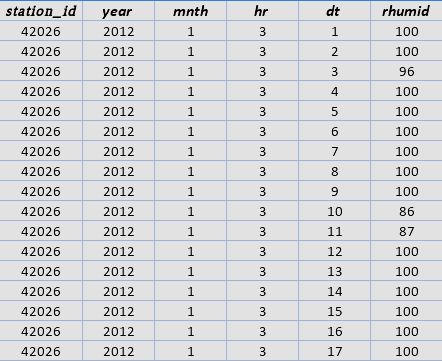Figure 2. Instances of relative humidity at 12 am and 3 pm

## 3.png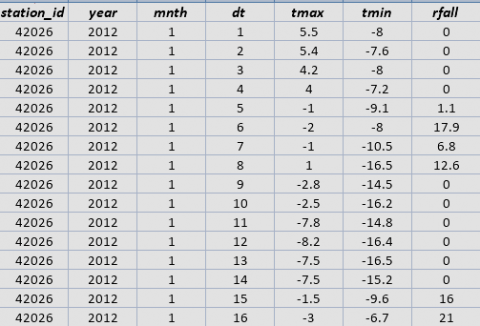Figure 3. Instances of maximum temperature, minimum temperature and rainfall

Furthermore, the two data files in Ref. , had been integrated into single complete dataset in which inconsistencies were resolved, cleaned & transformed and loaded to form a single dataset, shown below (Figure 4). This resultant integrated dataset contains data from year 2012 to 2017, for all the three stations. The following attributes are:

• Maximum temperature (tmax).
• Minimum temperature (tmin).
• Rainfall (rfall).
• Humidity measured 12 AM (humid12) and 3 PM (humid3).

This above continuous valued attributes have been converted into discretized valued attributes on the basis of GINI index and the snapshot of the normalized resultant dataset is shown below (Figure 5).

## 4.png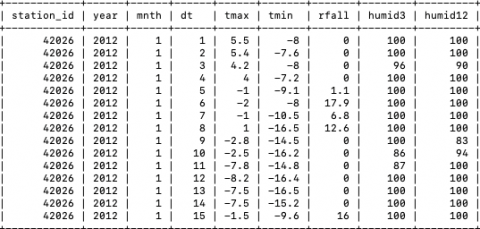Figure 4. Cleaned and integrated dataset

## 5.png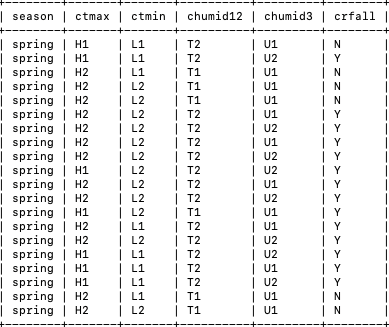Figure 5. Labeled resultant dataset

where,

Ctmax has been labeled into H1 and H2

Ctmin has been labeled into L1 and L2

Chumid12 has been labeled into T1 and T2

Chumid3 has been labeled into U1 and U2.

Also, the months have been splitted into seasons, shown below (Table 1) and the attribute crfall has been splitted into Y and N, if crfall is greater than 0 and less than 0 respectively [14, 15].

Table 1. Splitting months in respected seasons

 Season Months Winter 12, 1, 2 Spring 3, 4, 5 Summer 6, 7, 8 Autumn 9, 10, 11
4. Decision Tree Implementation

ID3, C4.5 and CART implement non-backtracking, greedy approach to build decision tree, constructed in top down approach following recursive divide and conquer methodology .

In this paper, we use Information Gain for selecting the attribute that best discriminates the given tuples into classes instead of Gini Index because Gini Index forces the resulting tree to be binary, while as there is no limitation with Information Gain.

4.1 Information gain

The information gain can be defined as the reduction in the entropy after the dataset is divided on an attribute. To calculate the information gain of an attribute a comparison of the entropy of the dataset after and before a transformation needs to be done. The attribute with the highest information gain will lead to the construction of a decision tree by acting as a splitting node with the homogenous branches.

Step 1: Calculate entropy of the target

 Rainfall Yes No 1407 2758 Total = 4165

$\operatorname{Info}(D)=-\sum_{\mathrm{i}=1}^{\mathrm{m}} \mathrm{pi} \log 2(\mathrm{pi})$                       (1)

${Info}(D)=-1407 / 4165 * {LOG} 2(1407 / 4165)- 2758 / 4165 * {LOG} 2\left(\frac{2758}{4165}\right)$

$\operatorname{Info}(D)=0.922712569$

Step 2: Calculate Entropy and Information Gain for each Attribute. (2) (3) (4) (5) (6) (7).

 Season Rainfall Yes No Spring 462 543 1005 Summer 431 622 1053 Autumn 203 878 1081 Winter 311 715 1026 1407 2758 4165

$\operatorname{Info}_{\text {Season }}(D)=\sum_{j=1}^{v} \frac{\left|D_{j}\right|}{|D|} * \operatorname{Info}(D)$                 (2)

$Info_{Season }(D)=\frac{1005}{4165}*\left(-\frac{462}{1005} * \operatorname{LOG} 2 (\frac{462}{1005})\frac{543}{1005} * \operatorname{LOG} 2\left(\frac{543}{1005}\right)\right)$

$+\frac{1053}{4165} *\left(-\frac{431}{1053} * \operatorname{LOG} 2\left(\frac{431}{1053}\right)-\frac{622}{1053} *\right.LOG2 \left.\left(\frac{622}{1053}\right)\right)+$

$\frac{1081}{4165} *\left(-\frac{203}{1081} * \operatorname{LOG} 2\left(\frac{203}{1081}\right)-\frac{878}{1081} * \operatorname{LOG} 2\left(\frac{878}{1081}\right)\right)+$

$\frac{1026}{4165} *\left(-\frac{311}{1026} * \operatorname{LOG} 2\left(\frac{311}{1026}\right)-\frac{715}{1026} * \operatorname{LOG} 2\left(\frac{715}{1026}\right)\right)$

$\operatorname{Info}_{\text {Season }}(D)=0.88583526$

$\operatorname{Gain}($ Season $)=\operatorname{Info}(D)-\operatorname{Info}_{\text {Season }}(D)$

$\operatorname{Gain}($ Season $)=0.03687731$                     (3)

 Max Temp Rainfall Yes No H1 397 484 881 H2 1010 2274 3284 1407 2758 4165

$\operatorname{Info}_{\operatorname{tmax}}(\mathrm{D})=\sum_{\mathrm{j}=1}^{\mathrm{v}} \frac{\left|\mathrm{D}_{\mathrm{j}}\right|}{|\mathrm{D}|} * \operatorname{Info}(\mathrm{D})$                    (4)

$\operatorname{Info}_{\text {tmax }}(\mathrm{D})=\frac{881}{4165} *\left(-\frac{397}{881} * \operatorname{LOG} 2\left(\frac{397}{881}\right)-\frac{484}{881} * \operatorname{LOG} 2\left(\frac{484}{881}\right)\right)+$

$\frac{3284}{4165} *\left(-\frac{1010}{3284} * \operatorname{LOG} 2\left(\frac{1010}{3284}\right)-\frac{2274}{3284} * \operatorname{LOG} 2\left(\frac{2274}{3284}\right)\right)$

$\operatorname{Info}_{\text {tmax }}(D)=0.912035266$

Gain $\left(\operatorname{tmax}\right)=\operatorname{Info}(D)-\operatorname{Info}_{\text {tmax }}(D)$

Gain $($ tmax $)=0.0106773$

 Min Temp Rainfall Yes No L1 308 809 1117 L2 1099 1949 3048 1407 2758 4165

$\operatorname{Info}_{\mathrm{tmin}}(\mathrm{D})=\sum_{\mathrm{j}=1}^{\mathrm{v}} \frac{\left|\mathrm{D}_{\mathrm{j}}\right|}{|\mathrm{D}|} * \operatorname{Info}(\mathrm{D})$                    (5)

$\mathrm{Info}_{\mathrm{tmin}}(\mathrm{D})=\frac{1117}{4165} *\left(-\frac{308}{1117} * \operatorname{LOG} 2\left(\frac{308}{1117}\right)-\frac{809}{1117} * \operatorname{LOG} 2\left(\frac{809}{1117}\right)\right)+\frac{3048}{4165} *$

$\left(-\frac{1099}{3048} * \operatorname{LOG} 2\left(\frac{1099}{3048}\right)-\frac{1949}{3048} * \operatorname{LOG} 2\left(\frac{1949}{3048}\right)\right)$

$\operatorname{Info}_{\operatorname{tmin}}(\mathrm{D})=0.004656635$

Gain $(\operatorname{tmin})=\operatorname{Info}(\mathrm{D})-\operatorname{Info}_{\operatorname{tmin}}(\mathrm{D})$

Gain $(\operatorname{tmin})=0.004656635$

 Humidity12 Rainfall Yes No T1 517 2054 2571 T2 890 704 1594 1407 2758 4165

$Info _{\text {humidity12 }}\,\,\,(\mathrm{D})=\sum_{\mathrm{j}=1}^{\mathrm{v}} \frac{\left|\mathrm{D}_{\mathrm{j}}\right|}{|\mathrm{D}|} * \operatorname{Info}(\mathrm{D})$                  (6)

$\mathrm{Info}_{\text {humidity12 }}\,\,\,(\mathrm{D})=\frac{2571}{4165} *\left(-\frac{517}{2571} * \operatorname{LOG} 2\left(\frac{517}{2571}\right)-\frac{2054}{2571} * \operatorname{LOG} 2\left(\frac{2054}{2571}\right)\right)+$

$\frac{1594}{4165} *\left(-\frac{890}{1594} * \operatorname{LOG} 2\left(\frac{890}{1594}\right)-\frac{704}{1594} * \operatorname{LOG} 2\left(\frac{704}{1594}\right)\right)$

$Info_{\text {humidity }12}\,\,\, (D) =0.825923561$

$\text{Gain}(\text{humidity12})=\operatorname{Info}(D)-\operatorname{Info}_{\text {humidity } 12}\,\,\, (D)$

$\text{Gain}(\text{humidity12})=0.09678901$

 Humidity3 Rainfall Yes No U1 747 2361 3108 U2 660 397 1057 1407 2758 4165 Gain= 0.08667994

$\operatorname{Info}_{\text {humidity3 }}\,\,(\mathrm{D})=\sum_{\mathrm{j}=1}^{\mathrm{v}} \frac{\left|\mathrm{D}_{\mathrm{j}}\right|}{|\mathrm{D}|} * \operatorname{Info}(\mathrm{D})$                      (7)

$\operatorname{Info}_{\text {humidity }3}\,\,\,(\mathrm{D})=\frac{3108}{4165} *\left(-\frac{747}{3108} * \operatorname{LOG} 2\left(\frac{747}{3108}\right)-\frac{2361}{3108} * \operatorname{LOG} 2\left(\frac{2361}{3108}\right)\right)+\frac{1057}{4165} *$

$\left(-\frac{660}{1057} * \operatorname{LOG} 2\left(\frac{660}{1057}\right)-\frac{397}{1057} * \operatorname{LOG} 2\left(\frac{397}{1057}\right)\right)$

Info$_{\text {humidity3 }}\,\,(\mathrm{D})=0.836032632$

Gain $($humidity3$)=\operatorname{Info}(D)-\operatorname{Info}_{\text {humidity}3}\,\,(D)$

Gain(humidity3) $=0.08667994$

4.2 Comparison of information gain

Humidity12 has the largest Information Gain, accordingly Humidity12 shall be decision node and same process shall be repeated for every generated node.

 Attribute Information Gain Season 0.03687731 Tmax 0.0106773 Tmin 0.004656635 Humidity12 0.09678901 Humidity3 0.08667994

For the decision tree creation, we have implemented a step wise process in which root nodes are decided based on the maximum information gain. i.e. the highest information gain, of all the attributes, will be selected as the root node. Below are the steps which are involved in the Decision tree creation.

Step 1: In this step we have determined the top most node i.e. root node, this is the starting point of the decision tree.

From the above (Figure 6) calculation, we find that humidity12 has the highest information gain. Thus, humidity12 will be the root node and the resultant tree is shown below (Figure 7).

## 6.png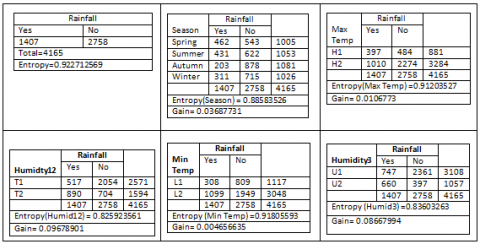Figure 6. Calculation of information gain

Step 2: We work from left to right; Calculations are performed for T2 value of humidity12 as shown below (Figure 8).

From the calculations shown above Humidity3 has the highest information gain accordingly. Thus attribute T2 on the basis of highest information gain value will be divided into U2 and U1, as shown below: (Figure 9).

## 7.png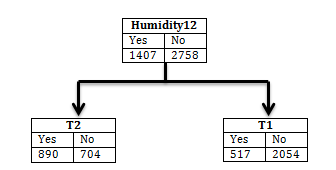Figure 7. Resultant tree on the basis of highest entropy

## 8.pngFigure 8. Calculation of information gain for T2

## 9.pngFigure 9. Resultant tree of T2 on the basis of highest entropy

Step 3: Here in this step, Calculations are performed for T2 value of humidity12 as shown below:

## 10.pngFigure 10. Calculation of information gain

From the calculations shown above (Figure 10) attribute season has the highest information gain accordingly. Thus attribute U2 on the basis of highest information gain value will be divided into Spring, Summer, Autumn and Winter, as shown below: (Figure 11).

These iterative processes continue until all the attributes (Humidity12, Humidity3, Maximum temperature, Minimum Temperature, season) are processed. After the completion of these iterative steps we came up with the tree representation of these given attributes, which is shown in the below (Figure 12).

## 11.png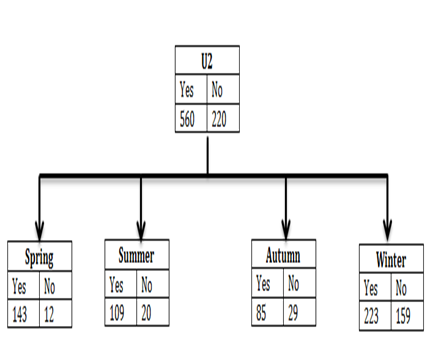Figure 11. Resultant tree of U2 on the basis of highest Entropy

## 12a.png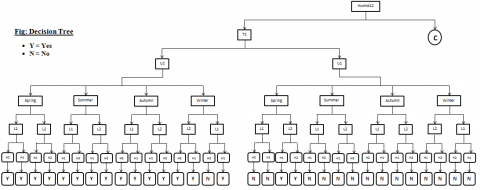## 12.png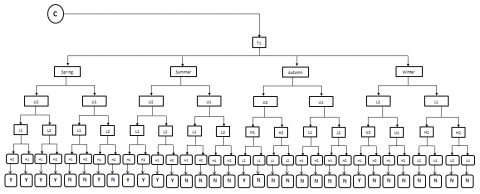Figure 12. Full decision tree

## 13.pngFigure 13. Pruned decision tree

5. MDL Pruning

A decision tree pruning has been performed in this paper by implementing Minimum Descriptive Length (MDL) approach. The MDL principle is a powerful inductive inference method that is the foundation of statistical modelling, pattern recognition, and machine learning. It holds that, based on the small set of known data, the best explanation is the one that allows the data to be compressed the most. In MDL a portion of a tree (sub-tree) is considered undependable and is therefore pruned if the Descriptive Length of the classification of the corresponding subsets of training instances together with the Descriptive Lengths of each path in the sub-tree is greater than the description length of the classification of the whole subset of training instances in the current node [26, 27]. Figure 13 shows pruned decision tree.

6.1. Working of gradient boosting algorithm

Figure 14 shows the flow process of gradient boosting in which the original data used for the prediction is passed to the base model where initial prediction is carried out. This prediction output is will be compared with the actual output and the error will be calculated. Based on the error the next decision tree is generated where only independent parameters are taken into consideration and for target parameter residuals are used (error).

The decision tree will predict another residue based on the previous error and the error is passed to the next residual model and this is a repetitive process until the error (residue) becomes nearer to zero. The final output will be calculated as the combination of the outputs of the base model and the individual outputs of the residual models. Thus in gradient boosting we are using a loss (residual) as an input to the next model and this process goes until we get the optimized model where we get error value close to zero.

Gradient boosting has same boosting hyper parameters by which we can tune our model and the accuracy will be much better than simple base model. In gradient boosting we don’t have a base estimator; we have by default decision tree as a base parameter. Also, the learning parameter will control the magnitude of the change. The learning rate ranges between 0 & 1, and it will make the model robust. The main advantage of the gradient boosting over simple base models is that it will not over fit and will allow us to generalize the model very well [28, 29].

After the implementation of Decision tree (ID3) on the geographical data, a booting approach is applied on the base model shown in Figure 14. This Original decision tree will be used as the basic model (Figure 15) where the residual (error) has been calculated and the error will be passed to the next level in which the independent parameters of the dataset are used to create the new model and the target parameter will be chosen as the error of the previous model [30, 31].

## 14.jpgFigure 14. Gradient boosting flow process

## 15.jpgFigure 15. Base model of gradient boosting

To construct the residual models in gradient boosting we need to calculate Log odds, Probability and Residuals.

So in order to calculate the log odds of the binary target class we need to apply the formula:

$\log o d d s=\log _{e} \frac{p}{1-p}$                     (8)

where, p denotes the number of particular classes in favor and this becomes the value for the initial leaf.

One of the easiest ways to use log (odds) for the classification is to convert it into the probability as shown below:

$\sigma=\frac{e^{\log (o d d s)}}{1+e^{\log (o d d s)}}$                    (9)

where, $\sigma$ is the probability and the threshold value for the probability distribution is 0.5 for the classification.

Computation of residuals is very important in the gradient boosting because in the entire tree construction instead of actual target parameter we use residuals as the decision parameter for the next iteration. So in order to calculate the residuals we need the existing class probability (observed) and the existing class labels (actual). Therefore, the residuals will be:

Residuals = Observed - Actual               (10)

Thus in order to implement gradient boosting on any base model we need to apply these steps iteratively until the residual error becomes nearer to zero. So the most common approach used in the implementation of the Gradient Boosting from the base model is:

$\frac{\sum Residual}{\sum\left[Prev_{probability} \quad \quad {*} \left(1- Prev _{Probablity} \quad\quad \right)\right]}$                     (11)

The final output of the gradient boosting thus follows the individual outputs of each residual model and the general approach is shown below:

$FinalOutput_{GradientBoosting} \quad = Output\,\, of\,\, Base\,\, Model +\gamma R M_{1}+\gamma R M_{2}+\cdots \gamma R$                   (12)

where, RM is the residual Model and $\gamma$ is the learning rate, which ranges between 0 to 1.

7. Performance

In this study, the researchers employed state-of-the-art technologies on geographical dataset with the prime purpose was to check the algorithm with the best overall performance and accuracy. In this paper, an approach has been made in which the step wise implementation of the decision tree on the geographical data has been proposed and after construction of original decision tree pruning has been applied on the same decision tree. Furthermore, a gradient boosting algorithm has been implemented on the dataset provided and the performance has been calculated. Here, we have shown the performance evaluation of all the three approaches sequentially as shown in below table (Table 2):

Table 2 shows a snapshot of results which includes accuracy, precision, recall values and many other calculations. The overall accuracy of the original tree is 81.50% in predicting the outcome class and approximately same accuracy is shown by the pruned decision tree. But as we can see that the accuracy value increases intensely to 87.5% when the gradient boosting is implemented on the same set of data where the original decision tree acts base estimator [32, 33].

Table 2. Accuracy statistics

 Specifications Decision Tree Gradient Boosting Without Pruning Pruned Test Set 1786 1786 1786 Correctly classified 1456 1448 1563 Wrong classified 330 338 223 Accuracy 0.815 0.811 0.875 Error 0.184 0.189 0.125 Recall (Rainfall) 0.502 0.484 0.108 Recall (No Rainfall) 0.945 0.944 0.952 Precision (Rainfall) 0.783 0.779 0.183 Precision(No Rainfall) 0.820 0.817 0.914 Cohen Kappa 0.497 0.482 0.073

## 16.pngFigure 16. Columnar representation of overall performance

## 17.png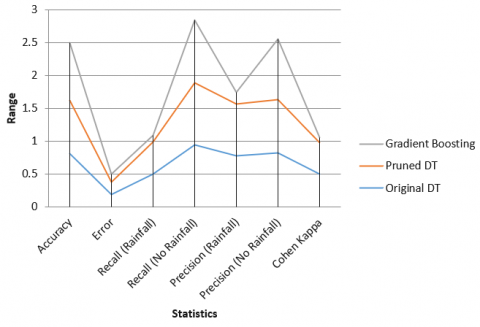Figure 17. Graphical representation of overall performance

The columnar (Figure 16) and graphical (Figure 17) representation of geographical data of Kashmir province is shown above, which helps in easy visualization of the results performed. Figure 16 and Figure 17 shows the comparative analysis of accuracy performance, error and other statistical measures when Gradient Boosting, Pruned Decision tree and original decision tree was implemented. It was observed that Gradient Boosting outperforms original decision tree and pruned decision tree performance with high precision rate of no rainfall.

Other methods are also very efficient method but they need a large portion of training data to train in order to predict very small portion of test data .

8. Conclusion and Future Work

In this paper decision tree is constructed from raw geographical data, this required conversion of continuous data attributes into discrete values, subsequently decision tree was pruned, performance was evaluated. It was observed that there is no significant increase in the performance when pruning was implemented. Furthermore, a simple gradient boosting algorithm has been implemented using same decision tree as a base estimator and the performance was calculated and it was observed that there is a drastic improvisation in accuracy and the accuracy measure got increased to 87.5% which is considered as the better performance as compared to basic decision tree performance.

Since gradient boosting shows better results but it takes time to compute the results, it is apt case for implementing XG-Boost and Cat-Boost algorithms on the said data set and compares its performance with the decision tree constructed in this paper.

References

 Agrawal, R., Srikant, R. (1994). Fast algorithms for mining association rules. In Proc. 20th Int. Conf. Very Large Data Bases, VLDB, 1215: 487-499.

 Zainudin, S., Jasim, D.S., Bakar, A.A. (2016). Comparative analysis of data mining techniques for Malaysian rainfall prediction. Int. J. Adv. Sci. Eng. Inf. Technol, 6(6): 1148-1153

 Fayaz, S.A., Zaman, M., Butt, M.A. (2021). An application of logistic model tree (LMT) algorithm to ameliorate Prediction accuracy of meteorological data. International Journal of Advanced Technology and Engineering Exploration, 8(84): 1424-1440. https://doi.org/10.19101/IJATEE.2021.874586

 Fayaz, S.A., Sidiq, S.J., Zaman, M., Ahmed, M. (2019). How machine learning is redefining geographical science: A review of literature. JETIR January, 6(1): 1731-1746.

 Tu, P.L., Chung, J.Y. (1992). A new decision-tree classification algorithm for machine learning. In TAI'92-Proceedings Fourth International Conference on Tools with Artificial Intelligence, 370-371. https://doi.org/10.1109/TAI.1992.246431

 Guyon, I., Elisseeff, A. (2003). An introduction to variable and feature selection. Journal of Machine Learning Research, 3: 1157-1182.

 Butt, M.A., Quadri, S.M.K., Zaman, M. (2012). Data warehouse implementation of examination databases. International Journal of Computer Applications, 44(5): 18-23. https://doi.org/10.5120/6259-8405

 Altaf, I., Butt, M.A., Zaman, M. (2022). Disease detection and prediction using the liver function test data: a review of machine learning algorithms. In International Conference on Innovative Computing and Communications, pp. 785-800. https://doi.org/10.1007/978-981-16-2597-8_68

 Fayaz, S.A., Zaman, M., Butt, M.A. (2022). Performance evaluation of Gini index and information gain criteria on geographical data: an empirical study based on JAVA and python. In International Conference on Innovative Computing and Communications, pp. 249-265. https://doi.org/10.1007/978-981-16-3071-2_22

 Fayaz, S.A., Zaman, M., Butt, M.A. (2021). To ameliorate classification accuracy using ensemble distributed decision tree (DDT) vote approach: An empirical discourse of geographical data mining. Procedia Computer Science, 184: 935-940. https://doi.org/10.1016/j.procs.2021.03.116

 Zaman, M., Butt, M.A. (2012). Information translation: A practitioners approach. In World Congress on Engineering and Computer Science (WCECS).

 Altaf, I., Butt, M.A., Zaman, M. (2021). A pragmatic comparison of supervised machine learning classifiers for disease diagnosis. In 2021 Third International Conference on Inventive Research in Computing Applications (ICIRCA), pp. 1515-1520. https://doi.org/10.1109/ICIRCA51532.2021.9544582

 Aswini, R., Kamali, D., Jayalakshmi. S., Rajesh, R. (2018). Predicting rainfall and forecast weather sensitivity using data mining techniques. International Journal of Pure and Applied Mathematics, 119(14): 843-847.

 Hemalatha, P. (2013). Implementation of data mining techniques for weather report guidance for ships using global positioning system. International Journal of Computational Engineering Research, 3(3): 198-202.

 Petre, E.G. (2009). A decision tree for weather prediction. Bul. Univ. Pet.–Gaze din Ploieşti, 61(1): 77-82.

 Ji, S.Y., Sharma, S., Yu, B., Jeong, D.H. (2012). Designing a rule-based hourly rainfall prediction model. In 2012 IEEE 13th International Conference on Information Reuse & Integration (IRI), pp. 303-308. https://doi.org/10.1109/IRI.2012.6303024

 Fayaz, S.A., Zaman, M., Butt, M.A. (2022). Knowledge discovery in geographical sciences-A systematic survey of various machine learning algorithms for rainfall prediction. In International Conference on Innovative Computing and Communications, pp. 593-608. https://doi.org/10.1007/978-981-16-2597-8_51

 Zaman, M., Kaul, S., Ahmed, M. (2020). Analytical comparison between the information gain and Gini index using historical geographical data. Int. J. Adv. Comput. Sci. Appl, 11(5): 429-440.

 Fayaz, S.A., Zaman, M., Butt, M.A. (2022). A hybrid adaptive grey wolf levenberg-marquardt (GWLM) and nonlinear autoregressive with exogenous input (NARX) neural network model for the prediction of rainfall. International Journal of Advanced Technology and Engineering Exploration.; 9(89): 509-522. https://doi.org/10.19101/IJATEE.2021.874647

 Fayaz, S.A., Altaf, I., Khan, A.N., Wani, Z.H. (2019). A possible solution to grid security issue using authentication: An overview. J. Web Eng. Technol, 5(3): 10-14.

 Mohd, R., Butt, M.A., Zaman, M. (2020). GWLM–NARX: Grey Wolf Levenberg–Marquardt-based neural network for rainfall prediction. Data Technologies and Applications, 54(1): 85-102. https://doi.org/10.1108/DTA-08-2019-0130

 Fayaz, S.A., Zaman, M., Kaul, S., Butt, M.A. (2022). Is deep learning on tabular data enough? An Assessment. International Journal of Advanced Computer Science and Applications (IJACSA), 13(4). http://dx.doi.org/10.14569/IJACSA.2022.0130454

 Fayaz, S.A., Zaman, M., Butt, M.A. (2022). Numerical and experimental investigation of meteorological data using adaptive linear M5 model tree for the prediction of rainfall. Review of Computer Engineering Research, 9(1): 1-12.

 Kaul, S., Fayaz, S.A., Zaman, M., Butt, M.A. (2022). Is decision tree obsolete in its original form? A Burning debate. Revue d'Intelligence Artificielle, 36(1): 105-113. https://doi.org/10.18280/ria.360112

 Kononenko, I. (1998). The minimum description length based decision tree pruning. In Pacific Rim International Conference on Artificial Intelligence, pp. 228-237. https://doi.org/10.1007/BFb0095272.

 Geetha, A., Nasira, G.M. (2014). Data mining for meteorological applications: Decision trees for modeling rainfall prediction. In 2014 IEEE International Conference on Computational Intelligence and Computing Research, pp. 1-4. https://doi.org/10.1109/ICCIC.2014.7238481

 Mohd, R., Butt, M.A., Zaman, M. (2022). Grey wolf-based linear regression model for rainfall prediction. International Journal of Information Technologies and Systems Approach (IJITSA), 15(1): 1-18. https://doi.org/10.4018/IJITSA.290004

 Hassan, M., Butt, M.A., Zaman, M. (2021). An Ensemble random forest algorithm for privacy preserving distributed medical data mining. International Journal of E-Health and Medical Communications (IJEHMC), 12(6), 1-23.

 Shehloo, A.A., Butt, M.A., Zaman, M. (2021). Factors affecting cloud data-center efficiency: A scheduling algorithm-based analysis. International Journal of Advanced Technology and Engineering Exploration 8(82): 1136-1167. https://doi.org/10.19101/IJATEE.2021.874313

 Ashraf, M., Ahmad, S.M., Ganai, N.A., Shah, R.A., Zaman, M., Khan, S.A., Shah, A.A. (2021). Prediction of cardiovascular disease through cutting-edge deep learning technologies: An empirical study based on TENSORFLOW, PYTORCH and KERAS. In International Conference on Innovative Computing and Communications, pp. 239-255. https://doi.org/10.1007/978-981-15-5113-0_18

 Mohd, R., Butt, M.A, Zaman, M. (2018). SALM-NARX: Self adaptive LM-based NARX model for the prediction of rainfall. In 2018 2nd International Conference on I-SMAC (IoT in Social, Mobile, Analytics and Cloud)(I-SMAC) I-SMAC (IoT in Social, Mobile, Analytics and Cloud)(I-SMAC), 2018 2nd International Conference on, pp. 580-585. https://doi.org/10.1109/I-SMAC.2018.8653747

 Ashraf, M., Zaman, M., Butt, M.A. (2018). Performance analysis and different subject combinations: an empirical and analytical discourse of educational data mining. In 2018 8th International Conference on Cloud Computing, Data Science & Engineering (Confluence), pp. 287-292.

 Mir, N.M., Khan, S., Butt, M.A., Zaman, M. (2016). An experimental evaluation of Bayesian classifiers applied to intrusion detection. Indian Journal of Science and Technology, 9(12): 1-7. https://doi.org/10.17485/ijst/2016/v9i12/86291

 Ashraf, M., Zaman, M., Ahmed, M. (2018). Using ensemble StackingC method and base classifiers to ameliorate prediction accuracy of pedagogical data. Procedia Computer Science, 132: 1021-1040. https://doi.org/10.1016/j.procs.2018.05.018